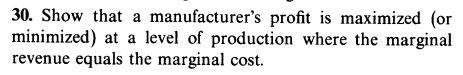# Min max problem

## Homework Statement## Homework Equations

marginal revenue[/B] (R') is the additional revenue that will be generated by increasing product sales by one unit

## The Attempt at a Solution

I don't know how to start. Q is the number of items sold at price x. y is the marginal cost, the cost of producing one item. N is the net profit, the revenue (my english isn't perfect so i explain each variable) ##~N=Q(x-y)~##.
The derivative N' is the marginal revenue, no?
Q, x and y are variables. x and y can change according to Q, because if i produce more i can lower the price i charge (x) and also the cost y.
How do i express marginal revenue?

#### Attachments

Homework Helper
Gold Member
Quantity sold/quantity produced ## Q=Q(P) ##, meaning ## Q ## is a function of ## P ##, where ## Q## is the quantity sold at price ##P ##. ## \\ ## Revenue ## R=Q \cdot P ##. ## \\ ## The cost to make ## Q ## items is a function of ## Q ##, so that ## C=C(Q) ##. ## \\ ## Net profit ## N=R-C ##. ## \\ ## Since ## Q=Q(P) ##, we can also write it in the form ## P=P(Q) ##, i.e. ## P ## is a function of ## Q ##. Thereby, revenue ## R=Q \cdot P=Q \cdot P(Q) ## is a function of ## Q ##, so we can write ## R=R(Q) ##. ## \\ ## We see that ## N=N(Q)=R(Q)-C(Q) ##. ## \\ ## How do we maximize ## N ##, using calculus? Also, what is the definition of marginal revenue, using calculus? And what is the definition of marginal cost, using calculus? ## \\ ## Once we find the ## Q ## where marginal revenue =marginal cost, we can then go to the function ## P=P(Q) ##, the inverse function of ## Q=Q(P) ##, to determine what price we should charge. The net profit ## N ## will be maximized at that selling price.

Last edited:
•Delta2
Marginal revenue=R':
$$R=Q \cdot P=Q \cdot P(Q)~\rightarrow~R'=P(Q)+Q\cdot P'(Q)$$
Marginal cost=C': ##~C=C(Q)~\rightarrow~C'=C'(Q)##
Marginal revenue=marginal cost: ##~P(Q)+Q\cdot P'(Q)=C'(Q)##
It doesn't help. C=C(Q) needn't be ##~C=Q\cdot k~##, the more items you make it's cheaper for each item.
And also, i have to prove that when Marginal revenue=marginal cost N is biggest

•